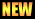MATH-300: Basic Mathematics for Chemist

Without mathematics the sciences cannot be understood, nor made clear, nor taught, nor learned. (Roger Bacon, 1214–1292)

Introdtuction; Review of basic algebra, Graphs and their significance in chemistry. Trigonometric, logarithmic and exponential functions. Differentiation, partial differentiation, differential equations and their use in chemical problems. Concept of maxima and minima. integration, Determinants and Matrices, their properties and use in chemical problems. solutions of linear equations (simple, determinant and matrices methods), operator theory, The eigen value problems and curve fitting.

Sample Problems 1
• A number is a mathematical object used to count, label, and measure. In mathematics, the definition of number has been extended over the years to include …
• In mathematics, the factorial of a non-negative integer n, denoted by n!, is the product of all positive integers less than or equal to n. For example,. 5 ! = 5 \times 4 …
• In mathematics, a function is a relation between a set of inputs and a set of permissible outputs with the property that each input is related to exactly one output.
• Graph 4.4.2 (Easy to use software to draw graphs)
• Trigonometric Values Handout (by Khalid Mehmood)
• Mid Term Result1. Paul M. “Maths for Chemistry” Edition 1st, Oxford University Press, UK (2006).
2. Ghram D. “Mathematics in Chemistry” Edition 1st, Prentice Hall, NY (1996).
3. Tebutt P. “Basic Mathematics for Chemist” 2nd Edition. John Wiley & Sons, NY (1998).
• atiq/math-300